﻿ 大数据驱动和分析的舰船通信网络流量智能估计
 舰船科学技术2022, Vol. 44Issue (10): 123-126    DOI: 10.3404/j.issn.1672-7649.2022.10.025PDF

Intelligent traffic estimation of ship communication network driven and analyzed by big data
MENG Xiang-rui
College of Applied Technology, Dalian Ocean University, Dalian 116300, China
Abstract: Taking the accurate estimation of ship communication network traffic as the research core, an intelligent estimation method of ship communication network traffic based on big data driving and analysis is proposed. This method takes empirical mode decomposition and convolution neural network in the field of big data driving and analysis as the technical core, and uses the signal denoising method of ship communication network based on improved ensemble empirical mode decomposition to remove the noise in the signal of ship communication network. After constructing the traffic time series diagram in the denoised ship communication network signal and highlighting the change characteristics of the communication network traffic in each time period, take the time series in the ship communication network traffic time series diagram as the prediction sample of the network traffic prediction method based on convolution neural network, extract the time series of the ship communication network detail signal, and estimate the trend information and time information of the traffic, Realize the intelligent estimation of ship communication network traffic. The test shows that the estimation result is basically consistent with the actual traffic after the intelligent estimation of ship communication network traffic by this method.
Key words: big data driven     worship     signal communication     network     flow     intelligent estimation
0 引　言

1 基于大数据驱动的舰船通信网络流量估计方法 1.1 改进集合经验模态分解的舰船通信网络信号降噪方法

 $d\left( j \right) = \sum\limits_{i = 1}^M {x\left( m \right){v_{1j}}\left( m \right)} /\sqrt {\sum\limits_{i = 1}^M {{x^2}\left( m \right)\sum\limits_{i = 1}^M {v_{1j}^2\left( m \right)} } }。$ (1)

 $h_\delta ^* = \max \left\{ {{h_\delta }\left| {{\beta _{2j}} < {\varepsilon _\delta }} \right.} \right\}{v_{2j}}\left( m \right)，$ (2)
 ${\varepsilon _\delta } = 0.56{v_{2j}}\left( m \right) - 0.2{\beta _{2j}}{v_{2j}}\left( m \right) 。$ (3)

 $x'\left( m \right) = r\left( m \right){\varepsilon _\delta } + \sum\limits_{j = p}^{{N_1} - 1} {{v_{1j}}\left( m \right){\varepsilon _\delta }} + {s_{{N_1}}}\left( m \right){\varepsilon _\delta }。$ (4)

1.2 舰船通信网络流量时间序列图的构建

 $R\left( S \right) = - x'\left( m \right)\sum\limits_{j = 1}^m {Q_j^2}。$ (5)

1）点的描述。把信息熵信息实施归一化，把其数值映射至0~1之间，之后结合映射后数值分解成4种级别，级别信息如表1所示。将源/目的IP、源/目的端口的4个点依次设成 ${A_1}$ ${A_2}$ ${B_1}$ ${B_2}$ ，信息熵数值的级别是 $i$ $i$ 对应表1中的级别信息。某时刻舰船通信网络流量采样点中属性1、属性2的信息熵模式依次是 ${A_{1i}}$ ${A_{2i}}$ ${B_{1i}}$ ${B_{2i}}$表 1 映射数值级别 Tab.1 Mapping value level

2）边的描述。设置 $t$ 时间段中属性1、属性2的信息熵依次是 ${R^t}\left( {{A_{1i}}} \right)$ ${R^t}\left( {{A_{2i}}} \right)$ ${R^t}\left( {{B_{1i}}} \right)$ ${R^t}\left( {{B_{2i}}} \right)$ 。设置2个属性点的边权重为：

 ${\varpi ^t}\left( {a,b} \right) = \frac{{{R^t}\left( {{A_{1i}}} \right) - {R^t}\left( {{A_{2i}}} \right)}}{{{R^t}\left( {{B_{1i}}} \right) - {R^t}\left( {{B_{2i}}} \right)}}。$ (6)

1.3 基于卷积神经网络的网络流量预测方法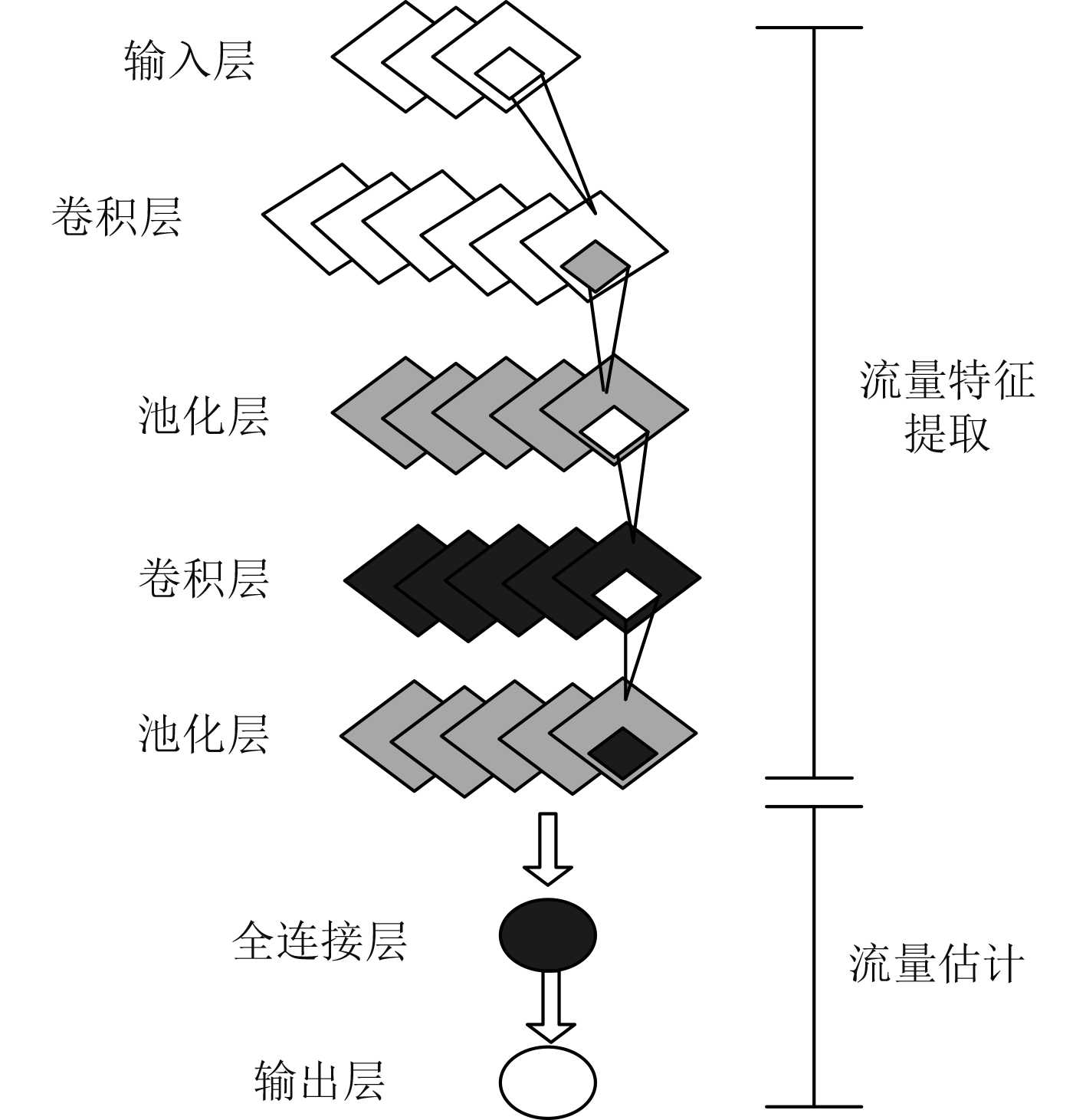图 1 卷积神经网络结构图 Fig. 1 Structure diagram of convolutional neural network

 $S\left( k \right) = \frac{{F\left[ {\left( {{Y_t} - {\lambda _t}} \right)\left( {{Y_{t + h}} - {\lambda _{t + h}}} \right)} \right]}}{{{\theta ^2}}} 。$ (7)

 $M = \sqrt {n + m} + b 。$ (8)

 $Y\left( t \right) = \sum\limits_{i = 0}^i {{E_i}\left( t \right)} {\text{ + }}{B_i}\left( t \right) 。$ (9)

2 实验结果与分析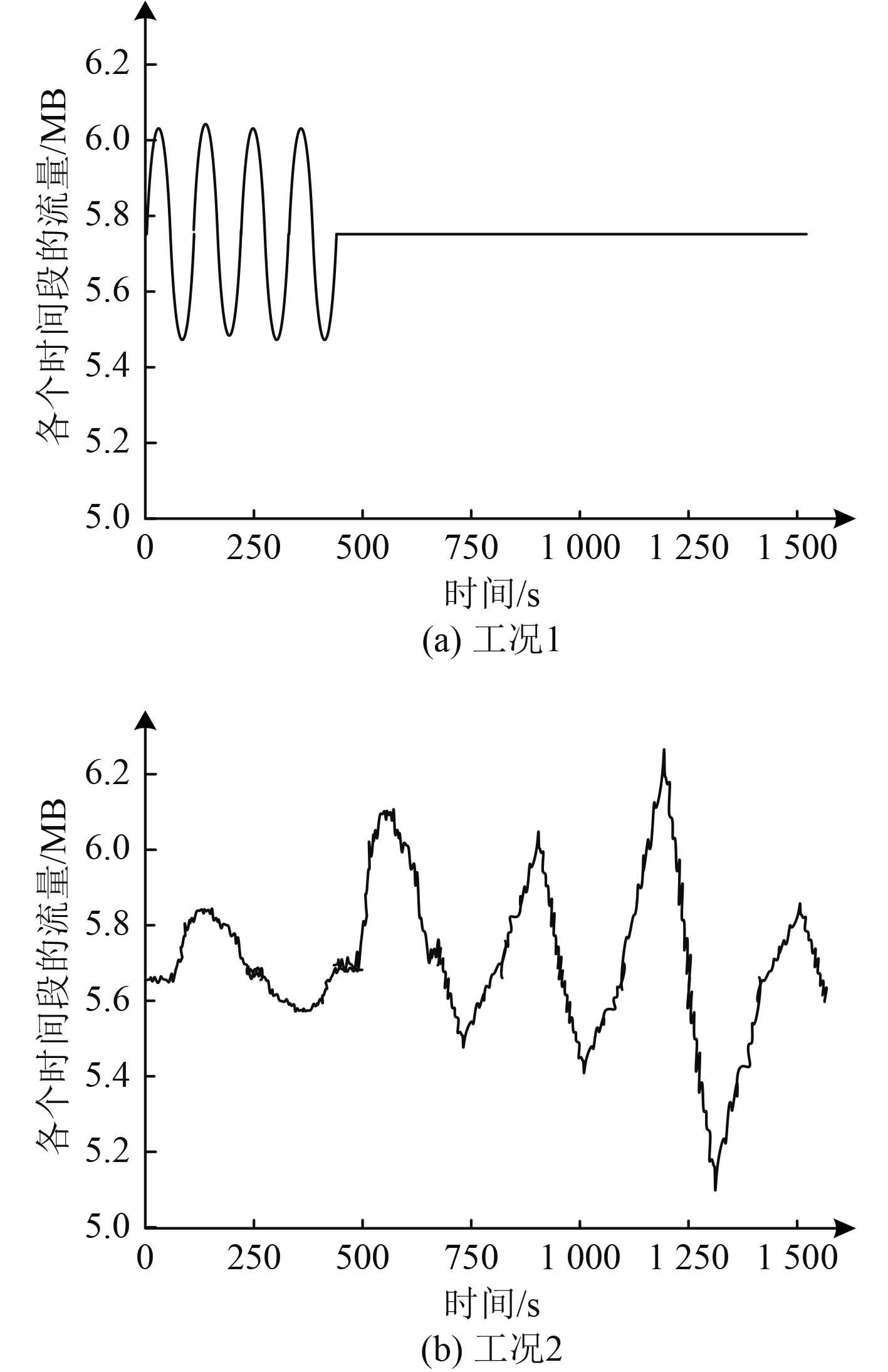图 2 某舰船通信链路不同时间粒度的网络流量数据 Fig. 2 Network traffic data of a ship communication link with different time granularity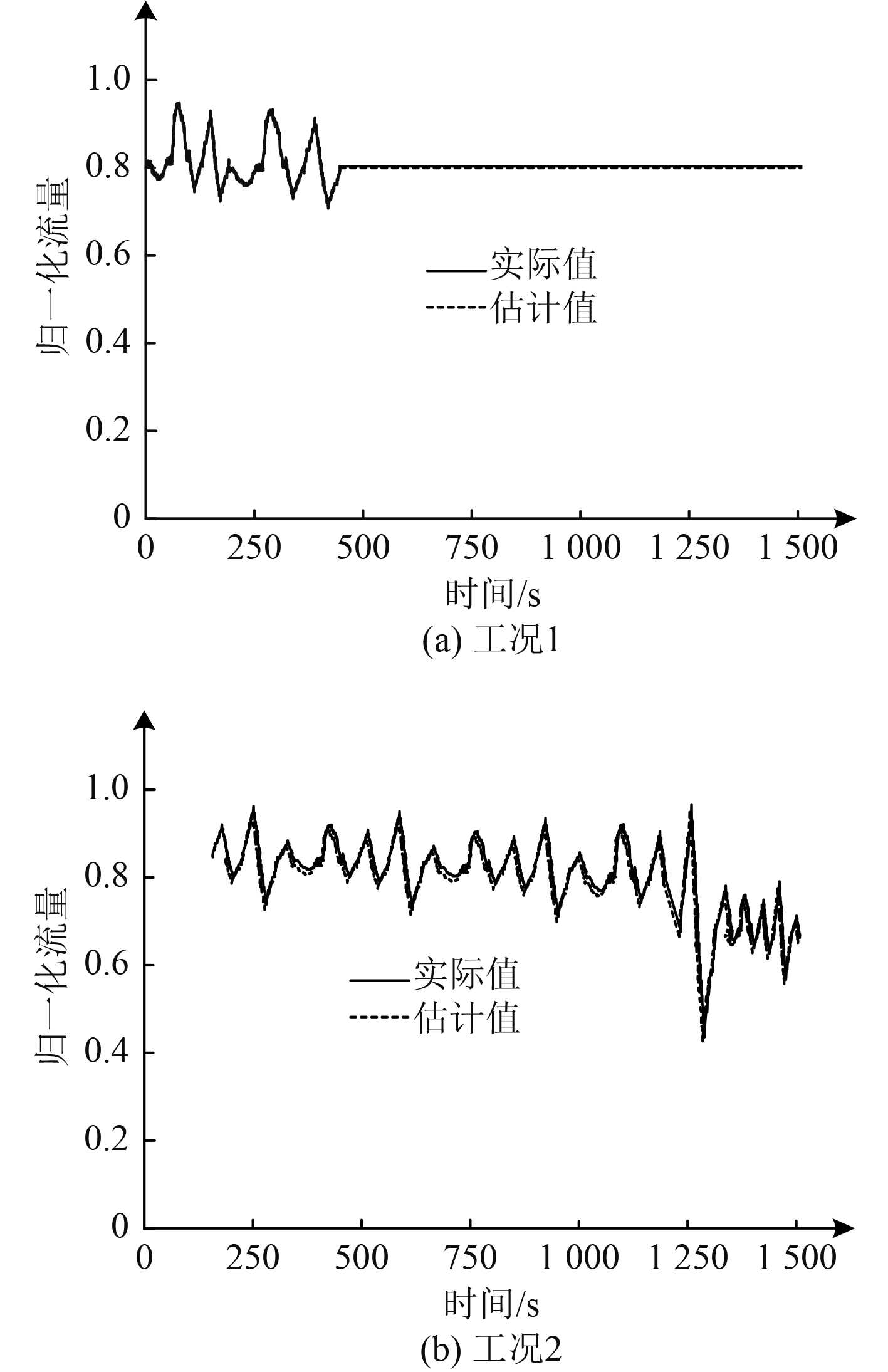图 3 本文方法对舰船通信网络流量估计结果 Fig. 3 This method is used to estimate the traffic of ship communication network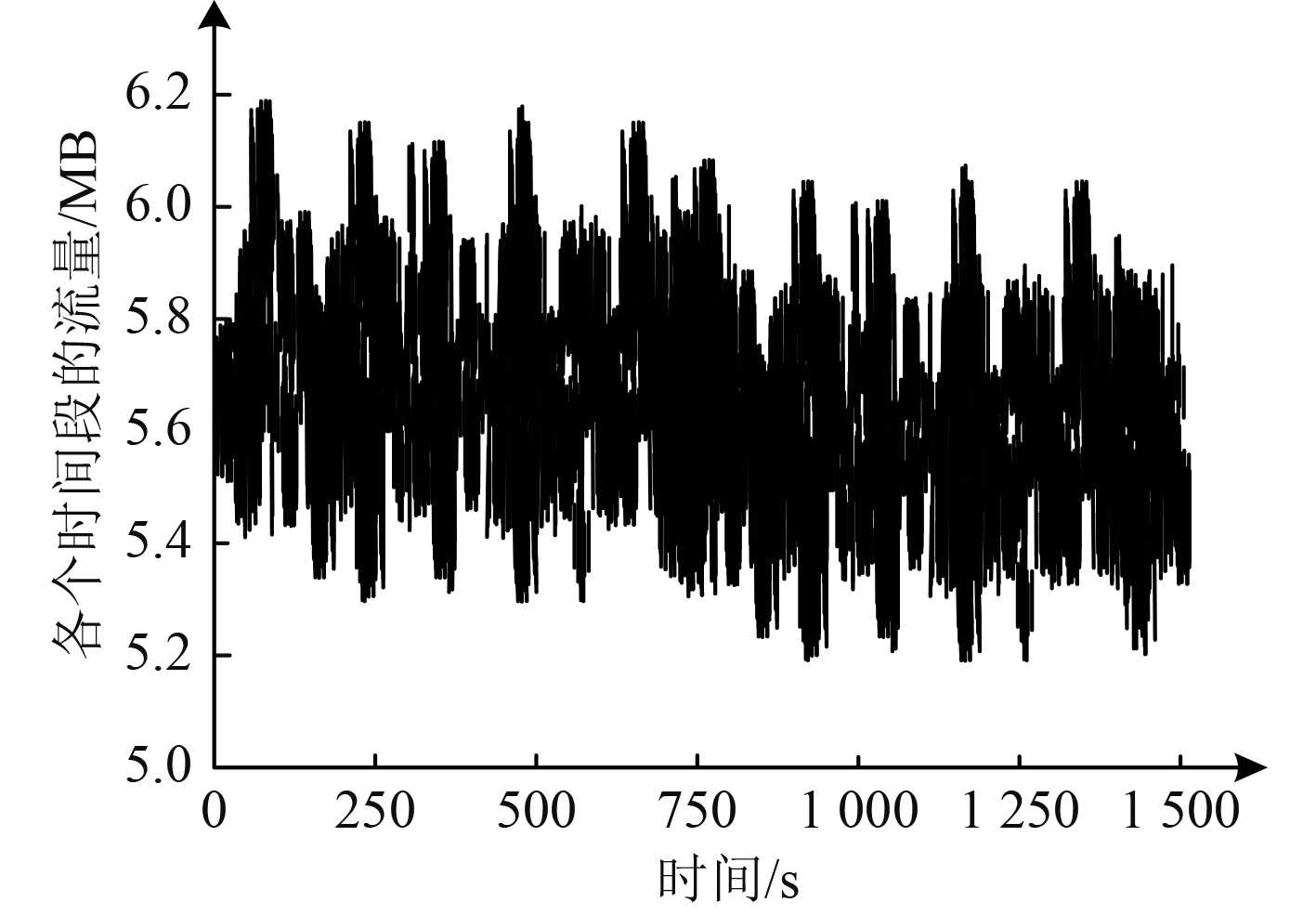图 4 加入噪声的舰船通信网络流量 Fig. 4 Ship communication network traffic with noise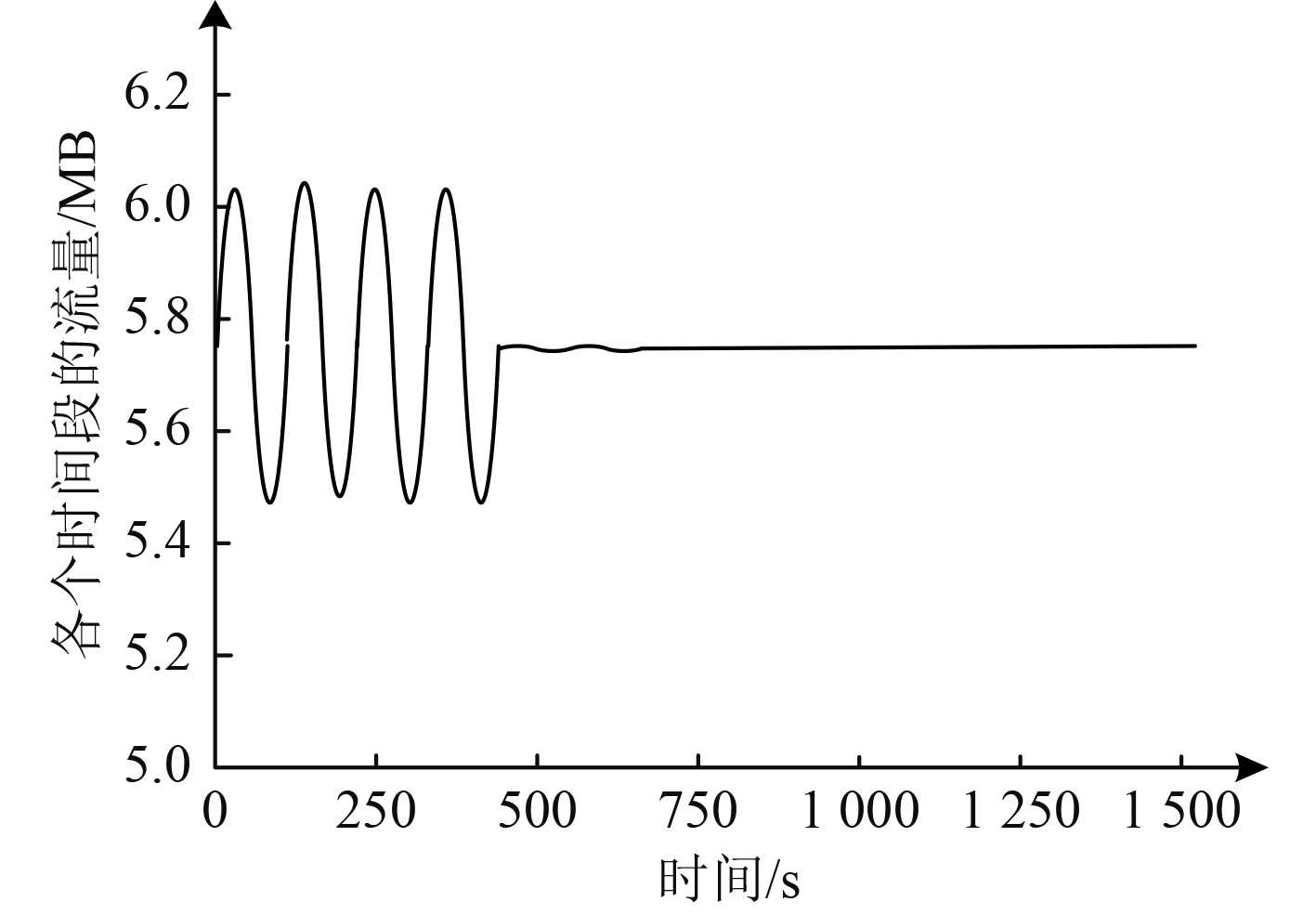图 5 本文方法处理后的舰船通信网络流量 Fig. 5 The ship communication network traffic processed by this method
3 结　语

  徐轶群, 徐弘, 孟令超, 等. 全船无线通信系统网络架构与可靠性研究[J]. 船舶工程, 2021, 43(6): 85-89+95.  周毅. 智能船舶网络风暴测试及抑制技术[J]. 船海工程, 2021, 50(3): 41-44+48. DOI:10.3963/j.issn.1671-7953.2021.03.011  金华标, 肖骁. 基于北斗短报文与4G的内河船载智能终端船岸通信技术[J]. 船海工程, 2021, 50(4): 67-71+76. DOI:10.3963/j.issn.1671-7953.2021.04.015  晓敏. 改进灰狼算法优化支持向量机的网络流量预测[J]. 电子测量与仪器学报, 2021, 35(3): 211-217.  郭佳, 余永斌, 杨晨阳. 基于全注意力机制的多步网络流量预测[J]. 信号处理, 2019, 35(5): 758-767.  曹素娥, 杨泽民. 基于聚类分析算法和优化支持向量机的无线网络流量预测[J]. 计算机科学, 2020, 47(8): 319-322.  陈浩杰, 黄锦, 左兴权, 等. 基于宽度&深度学习的基站网络流量预测方法[J]. 郑州大学学报(工学版), 2022, 43(1): 7-13.  郭芳, 陈蕾, 杨子文. 基于MGU的大规模IP骨干网络实时流量预测[J]. 山东大学学报(工学版), 2019, 49(2): 88-95.  张杰, 白光伟, 沙鑫磊, 等. 基于时空特征的移动网络流量预测模型[J]. 计算机科学, 2019, 46(12): 108-113. DOI:10.11896/jsjkx.181102207  戴宏亮, 罗裕达. 基于蝙蝠算法优化反向传播神经网络模型的无线网络流量预测[J]. 计算机应用, 2021, 41(S1): 185-188.  许绘香, 曹敏, 马莹莹. 基于大数据分析的非线性网络流量组合预测模型[J]. 沈阳工业大学学报, 2020, 42(6): 670-675.  李校林, 吴腾. 基于PF-LSTM网络的高效网络流量预测方法[J]. 计算机应用研究, 2019, 36(12): 3833-3836.